BetMGM 2019 Championship Odds
+300
3 to 1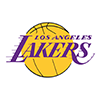Lakers
18.7% implied probability

+310
3.1 to 1Bucks
18.3% implied probability

+333
3.33 to 1Clippers
17.3% implied probability

+900
9 to 1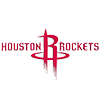Rockets
7.5% implied probability

+1000
10 to 176ers
6.8% implied probability

+1400
14 to 1Jazz
5.0% implied probability

+1500
15 to 1Nuggets
4.7% implied probability

+1800
18 to 1Celtics
3.9% implied probability

+2200
22 to 1Mavericks
3.3% implied probability

+2500
25 to 1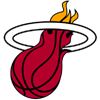Heat
2.9% implied probability

+3000
30 to 1Raptors
2.4% implied probability

+4000
40 to 1Pacers
1.8% implied probability

+5500
55 to 1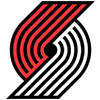Trail Blazers
1.3% implied probability

+6000
60 to 1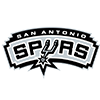Spurs
1.2% implied probability

No changes have been recorded yet.
+9000
90 to 1Nets
0.8% implied probability

+10000
100 to 1Thunder
0.7% implied probability

+12500
125 to 1Grizzlies
0.6% implied probability

+12500
125 to 1Magic
0.6% implied probability

+20000
200 to 1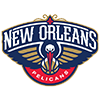Pelicans
0.4% implied probability

+25000
250 to 1Timberwolves
0.3% implied probability

+30000
300 to 1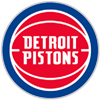Pistons
0.2% implied probability

+30000
300 to 1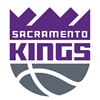Kings
0.2% implied probability

+35000
350 to 1Suns
0.2% implied probability

+50000
500 to 1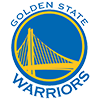Warriors
0.1% implied probability

+50000
500 to 1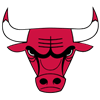Bulls
0.1% implied probability

+50000
500 to 1Hornets
0.1% implied probability

+75000
750 to 1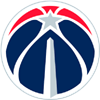Wizards
0.1% implied probability

+150000
1500 to 1Cavaliers
0.0% implied probability

+200000
2000 to 1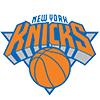Knicks
0.0% implied probability

+200000
2000 to 1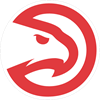Hawks
0.0% implied probability